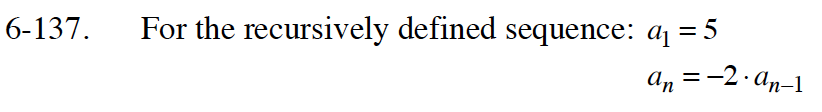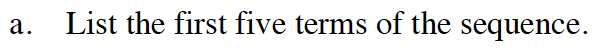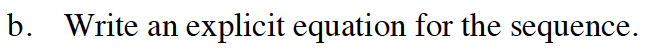Home > GB8I > Chapter cc46 > Lesson cc46.4.2 > Problem6-137

6-137.
1. For the recursively defined sequence: a1 = 5 Homework Help ✎
an = −2 · an−1

1. List the first five terms of the sequence.

2. Write an explicit equation for the sequence.Start by finding the second term in the sequence. Don't forget that the first term of the sequence is 5!

a2 = −2 · a2−1
a2 = −2 · a1
a2 = −2 · 5
a2 = −10

Repeat the above process for the next three terms of the sequence.What is the zeroth term?

What is the multiplier?

$a_{n}=-\frac{5}{2}(-2)^n$